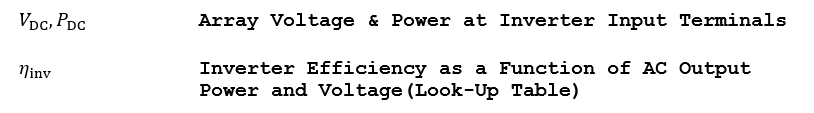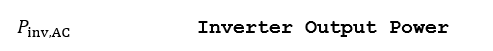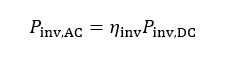# Inverter Efficiency

Compute the inverter output power by applying the inverter efficiency function. The efficiency table lists the inverter’s efficiency as a function of the input power, and at many DC input voltages, as shown in Figure 42.### Figure 42: Example Inverter Efficiency Curves Defined at Low, Medium, and High Voltages

Compute power at the output of the inverter by interpolating the efficiency curve, first by power, then by voltage. An interpolation technique which eliminates any overshoots and ripples, such as a cubic Hermite interpolation, is best, although a linear interpolation will suffice as a first approximation. A linear interpolation (with extrapolation for voltages less than the minimum or greater than the maximum) should be used for the voltages.

PlantPredict currently uses a trilinear interpolation across power and voltage and can support an arbitrary number of efficiency curves, expressed as percentage of AC power at a given input voltage. There is no physical limit on the number of efficiency points per voltage, although typically 3 are provided: one at minimum MPPT voltage, one at nominal voltage, and one at maximum MPPT voltage; furthermore any arbitrary “smoothness” can be achieved by interpolating the CEC, inverter datasheet or factory-witness test efficiency curves using a pre-processed non-linear interpolation.

## Inputs## Outputs## Algorithm

1.) Compute the inverter output power by linearly interpolating the efficiency curve at the DC input power.